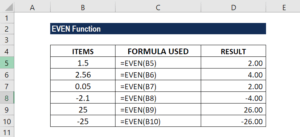# EVEN Function

Rounds a specified number away from zero to the next even number

## What is the EVEN Function?

The EVEN Function is classified as an Excel Math and Trigonometry function. It will round a specified number away from zero to the next even number. The function will round a positive number up and a negative number down.

As a financial analyst, EVEN is a very useful function, as it helps round a number and makes it an even number. It eliminates the least significant digits, simplifying the notation but keeping close to the original value. The function was introduced in MS Excel 2000.

### Formula

=EVEN(number)

The EVEN function uses the following argument:

1. Number (required argument) – This is the value we wish to round to the nearest even number.

### How to use the EVEN Function in Excel?

To understand the uses of the function, let’s consider an example:

#### Example

Suppose we are given the following data:

ITEMSFORMULAREMARKS
1.5 =EVEN(B5)Round up to the nearest even integer
2.56 =EVEN(B6)Round up to 4 and skips 3 as it is not an even integer
0.05 =EVEN(B7)Round up to 2 and skips 1 as it is not an even integer
-2.1 =EVEN(B8)Round up away from 0 and skips 3 as it is not an even integer
25 =EVEN(B9)Round up to 26, the next even integer
-25 =EVEN(B10)Round up to -26, that is away from zero

The results are below:### Things to remember about the EVEN Function

1. #VALUE! error – Occurs when the supplied number argument is not recognized as a numeric value by Excel.
2. As the EVEN function always rounds numbers up, which is away from zero, a positive number will become larger and negative number will actually become a smaller number that is more negative.

Thanks for reading CFI’s guide to important Excel functions! By taking the time to learn and master these Excel functions, you’ll significantly speed up your financial analysis. To learn more, check out these additional CFI resources:

• Excel Functions for Finance